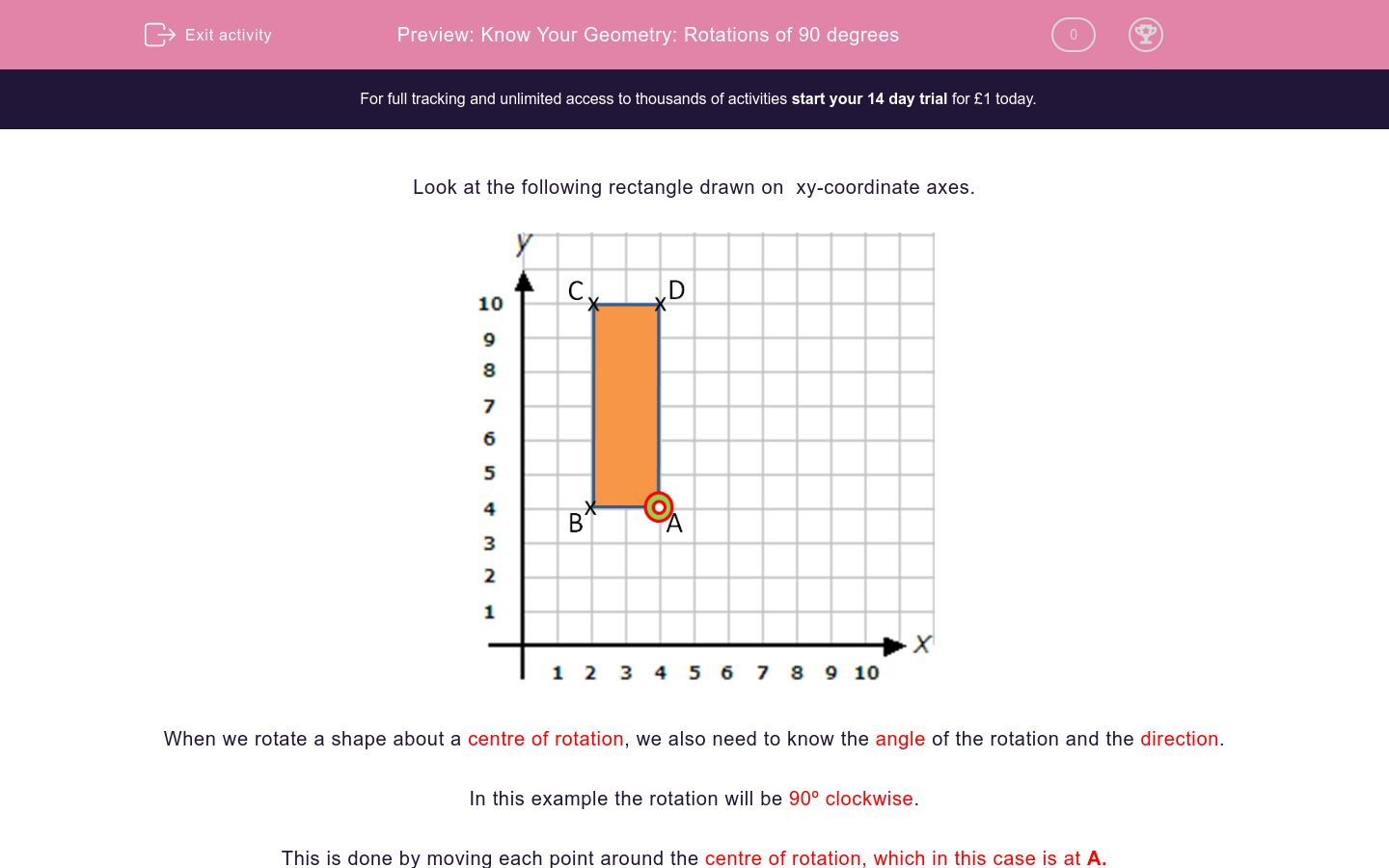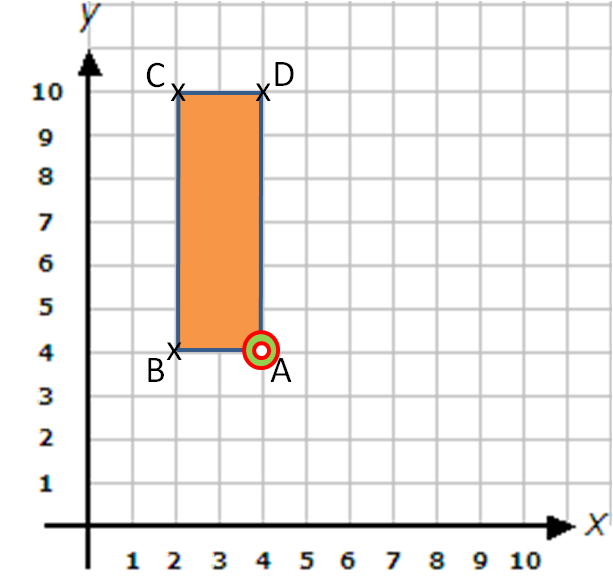# Know Your Geometry: Rotations of 90 degrees

In this worksheet, students rotate points and shapes 90º clockwise or anticlockwise around the given points and give the coordinates.Key stage:  KS 2

Curriculum topic:   Maths and Numerical Reasoning

Curriculum subtopic:   2D Shapes: Circles, Angles and Bearings

Difficulty level:### QUESTION 1 of 10

Look at the following rectangle drawn on  xy-coordinate axes.When we rotate a shape about a centre of rotation, we also need to know the angle of the rotation and the direction.

In this example the rotation will be 90º clockwise.

This is done by moving each point around the centre of rotation, which in this case is at A.

The result looks like this:Notice that A and A' are at the same point and have not moved.

Look at this diagram, which shows a rectangle ABCD, which is rotated through 90º clockwise about the point A to give the rectangle A'B'C'D'.

Write down the coordinates of B'. (Remember to write your answer in brackets).Look at this diagram, which shows a rectangle ABCD, which is rotated through 90º clockwise about the point A to give the rectangle A'B'C'D'.

Write down the coordinates of C'. (Remember to write your answer in brackets).Look at this diagram, which shows a rectangle ABCD, which is to be rotated through 90º clockwise about the point A to give the rectangle A'B'C'D'.

Write down the coordinates of D'. (Remember to write your answer in brackets).Look at this diagram, which shows a rectangle ABCD, which is to be rotated through 90º clockwise about the point A to give the rectangle A'B'C'D'.

Write down the coordinates of A'.Look at this diagram, which shows a rectangle ABCD, which is to be rotated through 90º clockwise about the point B to give the rectangle A'B'C'D'.

Write down the coordinates of B'.Look at this diagram, which shows a rectangle ABCD, which is to be rotated through 90º clockwise about the point B to give the rectangle A'B'C'D'.

Write down the coordinates of A'.Look at this diagram, which shows a rectangle ABCD, which is to be rotated through 90º clockwise about the point B to give the rectangle A'B'C'D'.

Write down the coordinates of C'.Look at this diagram, which shows a rectangle ABCD, which is to be rotated through 90º clockwise about the point B to give the rectangle A'B'C'D'.

Write down the coordinates of D'.Look at this diagram, which shows a rectangle ABCD, which is to be rotated through 90º anticlockwise about the point D to give the rectangle A'B'C'D'.

Write down the coordinates of C'.Look at this diagram, which shows a rectangle ABCD, which is to be rotated through 90º anticlockwise about the point D to give the rectangle A'B'C'D'.

Write down the coordinates of B'.• Question 1

Look at this diagram, which shows a rectangle ABCD, which is rotated through 90º clockwise about the point A to give the rectangle A'B'C'D'.

Write down the coordinates of B'. (Remember to write your answer in brackets).(4,6)
(4, 6)
• Question 2

Look at this diagram, which shows a rectangle ABCD, which is rotated through 90º clockwise about the point A to give the rectangle A'B'C'D'.

Write down the coordinates of C'. (Remember to write your answer in brackets).(10,6)
(10, 6)
• Question 3

Look at this diagram, which shows a rectangle ABCD, which is to be rotated through 90º clockwise about the point A to give the rectangle A'B'C'D'.

Write down the coordinates of D'. (Remember to write your answer in brackets).(10,4)
(10, 4)
• Question 4

Look at this diagram, which shows a rectangle ABCD, which is to be rotated through 90º clockwise about the point A to give the rectangle A'B'C'D'.

Write down the coordinates of A'.(4,4)
(4, 4)
• Question 5

Look at this diagram, which shows a rectangle ABCD, which is to be rotated through 90º clockwise about the point B to give the rectangle A'B'C'D'.

Write down the coordinates of B'.(2,4)
(2, 4)
• Question 6

Look at this diagram, which shows a rectangle ABCD, which is to be rotated through 90º clockwise about the point B to give the rectangle A'B'C'D'.

Write down the coordinates of A'.(2,2)
(2, 2)
• Question 7

Look at this diagram, which shows a rectangle ABCD, which is to be rotated through 90º clockwise about the point B to give the rectangle A'B'C'D'.

Write down the coordinates of C'.(8,4)
(8, 4)
• Question 8

Look at this diagram, which shows a rectangle ABCD, which is to be rotated through 90º clockwise about the point B to give the rectangle A'B'C'D'.

Write down the coordinates of D'.(8,2)
(8, 2)
• Question 9

Look at this diagram, which shows a rectangle ABCD, which is to be rotated through 90º anticlockwise about the point D to give the rectangle A'B'C'D'.

Write down the coordinates of C'.(4,8)
(4, 8)
• Question 10

Look at this diagram, which shows a rectangle ABCD, which is to be rotated through 90º anticlockwise about the point D to give the rectangle A'B'C'D'.

Write down the coordinates of B'.(10,8)
(10, 8)
---- OR ----

Sign up for a £1 trial so you can track and measure your child's progress on this activity.

### What is EdPlace?

We're your National Curriculum aligned online education content provider helping each child succeed in English, maths and science from year 1 to GCSE. With an EdPlace account you’ll be able to track and measure progress, helping each child achieve their best. We build confidence and attainment by personalising each child’s learning at a level that suits them.

Get started